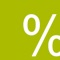# PerCent

価格 ｼﾞｬﾝﾙ サイズ このアプリは現在ストアで閲覧することができません。 120円 ダウンロードファイナンス 149.0KB HiveStudio ｼﾞｬﾝﾙ別：---総合：--- 2010-06-26 06:12:46 評価が取得できませんでした。 iOS 3.1.3以降が必要です。 iPhone、iPad および iPod touch 対応。 2022-08-13　このアプリは現在ストアから情報が取得できません。削除された可能性があります。
PerCent is an all-in-one percentage calculator. Its unique and simple interface will do any calculation you can imagine for you:

* add VAT to a price,
* compute discounts and sale prices,
* compute price before VAT or discount,
* compute the percentage discount,
* and more.

PerCent is the only app that will answer all your percentage related questions at once.

== How does it work? ==

In a percent calculation we always have four values:

* the original price (the price before taxes or discounts),
* the percentage rate to be applied,
* the price difference (either the taxes or the discount),
* the final price (the original price plus the price difference).

These values are presented in the application as an addition just as the one you would do in a paper. You can write any two of the values in the interface and the other two will be computed automagically for you. The calculation you see on the screen is always correct.

Let's see some examples:

Just write the price before tax in the original box, and the tax percentage in the percentage box. PerCent will instantly compute the amount of taxes in the difference box and the price after tax in the final box.

== Applying discounts ==

Write the price in the original box and the percentage discount in the percentage box. Tap on the plus sign and it will switch to a minus sign. The discount amount appears now in the difference box and the sale price in the final box.

== Taking tax off a price ==

Write the price after taxes in the final box and the tax percentage in the percentage box. The price before taxes appears now in the original box and the amount of taxes in the difference box.

== Finding the price before discount ==

Write the sale price in the final box and the percentage discount in the percentage box. Tap on the plus sign and it will switch to a minus sign. The price before the discount is now in the original box and the discount is in the difference box.

== Finding the percentage discount ==

Write the price in the original box and the sale price in the final box. The percentage discount and the price difference will automatically be computed.

== Sherlock Holmes calculations ==

Assume you know the discount and percentage discount that was applied to a price, but you don't know the price or the sale price. No problem. Write the info you have in the corresponding boxes and the prices will be computed for you.

Whichever two values you have, PerCent will find out the other two!

• 現在ランキング圏外です。ブログパーツ第二弾を公開しました！ホームページでアプリの順位・価格・周辺ランキングをご紹介頂けます。
ブログパーツ第2弾！

アプリの周辺ランキングを表示するブログパーツです。価格・順位共に自動で最新情報に更新されるのでアプリの状態チェックにも最適です。
ランキング圏外の場合でも周辺ランキングの代わりに説明文を表示にするので安心です。

サンプルが気に入りましたら、下に表示されたHTMLタグをそのままページに貼り付けることでご利用頂けます。ただし、一般公開されているページでご使用頂かないと表示されませんのでご注意ください。

オススメ！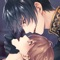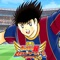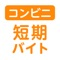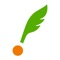お得情報ピックアップ！35%値引中50%値引中75%値引中66%値引中50%値引中Now loading...